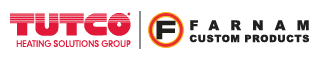Engineer Talk

# Estimating the Resistance Wire Temperature for an Open Coil Heating Element​For a typical convection air heating application, the air temperature and the volumetric air flow entering the heating element, and the power produced by the element are known or can be specified. The desired air temperature out of the heating element is achieved by commonly adjusting the power and/or the airflow. A first-order estimate of the air temperature leaving the element can be easily obtained using the formula below:

(1) P = m'cp(Tout−Tin)

Where:

P = power [Watts]

m' = mass flow rate [kg/s]1

cp = specific heat of air at Tavg[J/kg*K]

Tavg = (Tout−Tin)/2 [K]

Tout = output air temperature [K]

Tin = input air temperature [K]

Taking a quick initial look at the basic convection heat transfer equation below (sometimes referred to as Newton’s Law of Cooling), it appears it would be relatively straight forward to determine the resistance wire temperature of an open coil heating element.

(2) q = Q/A = h(Twire−Tair)

Where:

q = convective heat flux [W/m2]2

Q = heat dissipated by convection [Watts]

A = convective surface area [m2]

h = heat transfer coefficient [W/m2*K]3

Twire= resistance wire temperature [K]

Tair= air temperature [K]

However, in a typical basic heat transfer textbook (DeWitt, 1981), what’s not presented at the beginning of a section on convective heating, is that 'h' is not a simple constant independent of temperature. 'h' is typically calculated from an empirical formula4, dependent on temperature, air velocity, and geometry. A review of any basic heat transfer textbook will have a list of various formulas for h (or Nusselt number, Nu, a dimensionless constant from which h can be calculated) for different geometries. For our problem of the open coil heating element, a formula for a helix shape or geometry is typically not included.

A formula for forced convection against a coil was originally published by Cjnacki (Hegbom, 1997).  It is for coils with a Stretch Ratio ranging from 1.3 – 5, and is of the form:

(3) Nu = C = CRen

Where:

Re = Vd/v = Reynolds number5

Nu = hd/λ= Nusselt number

V = air velocity [m/s]; valid range 2-22 m/s

d = wire diameter [m]; valid range 1-4mm

v = kinematic viscosity of air [m2/s]

h = heat transfer coefficient [W/m2*K]

λ = thermal conductivity [W/m*K]

Twire = resistance wire temperature [°C]; valid range 200-800°C

Tair = air temperature [°C]; valid range 200-800°C

Solving for h yields:

(4) h = (λ/d)C(Vd)n

To determine c and n:

Te = (273+Twire)/(273+Tair)

lg(R1) = 3.368 − (0.278)Te

if < R1: C = [1.725](Te−3.328); n = 0.64 - (0.07)Te

if > R1: C = [1.84](Te−4.25); n = 0.74 - (0.07)Te

As the wire temperature increases, the heat dissipation due to radiation becomes more prominent and must be accounted for as well.

Power = I2R = Powerconvection + Powerradiation

For an open coil element, the radiation heat dissipation is undesirable, since the majority of the radiated heat is lost to the surroundings, and not into the airflow we are trying to heat.

Let’s now work through an example to show how the formula can be used to estimate wire temperature. We will assume radiation is negligible for this case.

For a given heater application we know:

Tair = 200°C

16 ga. resistance wire is used: d = .0508 in. = 1.291 mm

q = 30 W/in2

V = 15 m/s

With the ubiquitous availability of spreadsheet computing power, I found the most productive approach to be:

1. Estimate the wire temperature, Twire
2. Calculate the heat transfer coefficient using equation (4)
3. With equation (2), calculate q, the convective heat flux or watt density
4. Compare the difference with the known coil watt density. For the next iteration adjust the estimated wire temperature, Twire until the two values of q are approximately equal.

For the above conditions, iteration using a spreadsheet yielded the following result.

Estimated Calculated Known
Twire Watt Density, q[W/in2] Watt Density, q[W/in2]
400 40.97 30.00
375 35.69 30.00
350 30.46 30.00
349 30.25 30.00
348 30.04 30.00

The estimated resistance wire temperature = 348°C.

In conclusion, the above procedure can be used to estimate the resistance wire temperature of an open coil element when conditions are known to be predominately convective. This calculation is somewhat tedious even with the use of a spreadsheet, but once the spreadsheet is set up, future calculations are relatively straightforward. It also demonstrates the need for a thorough search of the literature for equations suitable for the conditions found with open coil elements. To date, I have not seen similar formulas that are applicable for open coil heating elements and would be delighted to hear from you to discuss, share information, thoughts regarding this. The development of more wide-ranging formulae overall would be of benefit to a wide range of industrial and commercial applications.

1Volumetric flow rate can be converted to a mass flow rate if the inlet air pressure (often p ≈ 1 atm) and the inlet air temperature are known.

2Convective heat flux is often referred to as the “watt density” with units of Watts/in2 in U.S.

3The heat transfer coefficient is sometimes referred to as the film coefficient as well.

4The implication being there is not a unified theory for convective heat transfer. Data is collected from experiment, and a formula is developed to fit the data

5Nu and Re are based on the characteristic length of wire diameter, d.

DeWitt, I. (1981). Fundamentals of Heat Transfer. New York: John Wiley & Sons, Inc.

Diepholz, D. (2013, January). SCFM, CFM What’s the difference? Retrieved July 18, 2013, from www.farnam-custon.com: http://www.farnam-custom.com/library/engineertalk/scfm-cfm-whats-the-difference

Hegbom, T. (1997). Integrating Electrical Heating Elements in Appliance Design. New York, New York: Marcel Dekker.

Tutco-Farnam Custom ProductsCalculators
Calculators +